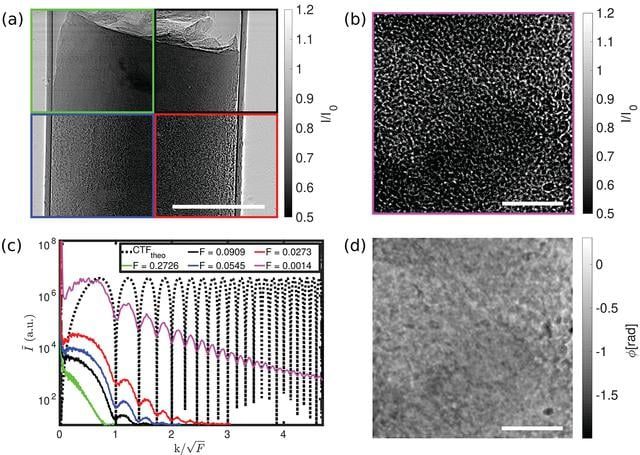disable zoom     view article Figure 4 (a) Empty-beam corrected projections of an unstained human pancreatic tissue sample (Sample A) for four different propagations distances for the parallel-beam illumination in the direct-contact regime. Green: x12 = 10 mm (F10 = 0.2726). Black: x12 = 30 mm (F30 = 0.0909). Blue: x12 = 50 mm (F50 = 0.0545). Red: x12 = 100 mm (F100 = 0.0273). Phase-contrast increases with decreasing Fresnel number. (b) Empty-beam corrected projection of the sample in (a) for the cone-beam illumination in the holographic regime. Magenta: x12 = 4975 mm (Fholo = 0.0014). (c) Power spectral densities of an squared area in the middle of the projected sample. The black dotted line shows the theoretical sinusoidal oscillations of the CTF. (d) CTF-reconstructed phase image of (b). Scale bars: (a) 500 µm and (b) and (d) 100 µm.JOURNAL OFSYNCHROTRONRADIATION
ISSN: 1600-5775
Volume 27| Part 6| November 2020| Pages 1707-1719